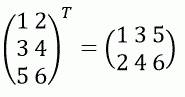Competitions

# Transpose the matrix

Given matrix A. Transpose it.

Let B be the transposed matrix A. Then Bij = Aji.#### Input

The first line contains the size of the matrix n and m (1n, m100). Each of the next n lines contains m integers and describe the matrix A.

#### Output

Print the transposed matrix A: m lines, each with n integers.

Time limit 1 second
Memory limit 128 MiB
Input example #1
3 2
1 2
3 4
5 6

Output example #1
1 3 5
2 4 6

Author Michael Medvedev